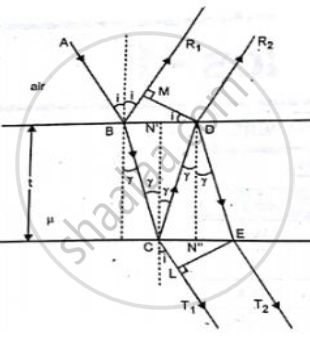# Obtain the condition for maxima and minima of the light reflected from a thin transparent film of uniform thickness. Why is the visibility of the fringe much higher in the reflected system - Applied Physics 2

Obtain the condition for maxima and minima of the light reflected from a thin transparent film of uniform thickness. Why is the visibility of the fringe much higher in the reflected system than in the transmitted system.

#### Solutioni. The two rays will interfere consecutively if the path difference between them is an integral multiple of λ i.e.
2μt cos r + λ/2 = nλ
Or
2μt cos r = (2n-1)λ/2
Where,
n= 1, 2, 3, 4, ……….. (for maxima)
or
2μt cos r = (2n-1)λ/2
Where n = 0, 1, 2, 3, 4, ………………….
When this condition is satisfied the film will appear bright in the reflected system.
ii. The two rays will interfere destructively if the path difference between them is an odd multiple of λ/2 i.e.
2μt cos r + λ/2 = (2n-1)λ/2
Or
2μt cos r = nλ (for minima)
iii. The film which appears bright in reflected light appears dark in transmitted light and vice versa.
iv. For the transmitted light, the intensity of maxima is about 100% and the minima is about 85%. The result in poor contrast between bright and dark

where in poor contrast between where as in reflected light, minima is having its intensity zero and maxima is nearly 15% of incident energy. This result in good contrast.
v. Hence visibility of fringe is much higher in reflected system.

Concept: Interference in Thin Film of Constant Thickness Due to Reflected and Transmitted Light
Is there an error in this question or solution?
2017-2018 (December) CBCGS

Share